# Computer Organization and Architecture - Online Test

Q1. A computer has a 256 KByte, 4-way set associative, write back data cache with block size of 32 Bytes. The processor sends 32 bit addresses to the cache controller. Each cache tag directory entry contains, in addition to address tag, 2 valid bits, 1 modified bit and 1 replacement bit. The number of bits in the tag field of an address is
Explaination / Solution: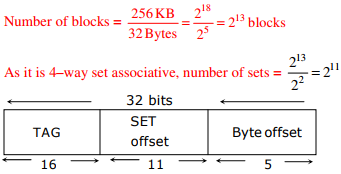Q2. A computer has a 256 KByte, 4-way set associative, write back data cache with block size of 32 Bytes. The processor sends 32 bit addresses to the cache controller. Each cache tag directory entry contains, in addition to address tag, 2 valid bits, 1 modified bit and 1 replacement bit. The size of the cache tag directory is
Explaination / Solution:

TAG controller maintains 16 + 4 = 20 bits for every block
Hence, size of cache tag directory = 20 × 213 bits =160 K bits

Q3. In a k-way set associative cache, the cache is divided into v sets, each of which consists of k lines. The lines of a set are placed in sequence one after another. The lines in set s are sequenced before the lines in set (s+1). The main memory blocks are numbered 0 onwards. The main memory block numbered j must be mapped to any one of the cache lines from
Explaination / Solution:

Position of main memory block in the cache (set) = (main memory block number) MOD (number of sets in the cache). As the lines in the set are placed in sequence, we can have the lines from 0 to (K – 1) in each set. Number of sets = v, main memory block number = j First line of cache = (j mod v)*k; last line of cache = (j mod v)*k + (k – 1)

Q4. The smallest integer than can be represented by an 8-bit number in 2’s complement form is
Explaination / Solution:Q5. A RAM chip has a capacity of 1024 words of 8 bits each (1K × 8) . The number of 2 × 4 decoders with enable line needed to construct a 16K × 16 RAM from 1K × 8 RAM is
Explaination / Solution:

RAM chip size = 1k × 8 [1024 words of 8 bits each]
RAM to construct = 16k × 16
Number of chips required = (16k × 16)/(1k × 8) = 16 × 2 [16 chips vertically with each having 2 chips horizontally]
So to select one chip out of 16 vertical chips, we need 4 x 16 decoder.
Available decoder is – 2 x 4 decoder
To be constructed is 4 x 16 decoder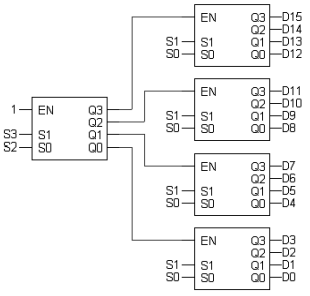So we need 5, 2 x 4 decoder in total to construct 4 x 16 decoder.

Q6. Consider an instruction pipeline with five stages without any branch prediction: Fetch Instruction (FI), Decode Instruction (DI), Fetch Operand (FO), Execute Instruction (EI) and Write Operand (WO). The stage delays for FI, DI, FO, EI and WO are 5 ns, 7 ns, 10 ns, 8 ns and 6 ns, respectively. There are intermediate storage buffers after each stage and the delay of each buffer is 1 ns. A program consisting of 12 instructions I1, I2 ,I3 ,......I12 is executed in this pipelined processor. Instruction I4 is the only branch instruction and its branch target is I9 . If the branch is taken during the execution of this program, the time (in ns) needed to complete the program is
Explaination / Solution:

Clock period=Maximum stage delay+ overhead (Buffer) =10+1=11 ns
Assume FI-1, DI-2, FO-3, EI-4, WO-5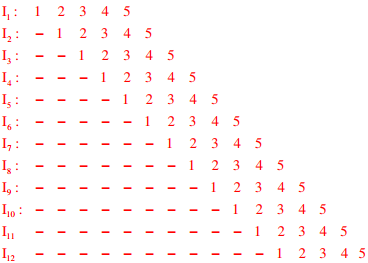So number of clocks required to complete the program is = 15 clocks and time taken is = 15 ×11 ns=165 ns.

Q7. The following figure represents access graphs of two modules M1 and M2. The filled circles represent methods and the unfilled circles represent attributes. IF method m is moved to module M2 keeping the attributes where they are, what can we say about the average cohesion and coupling between modules in the system of two modules?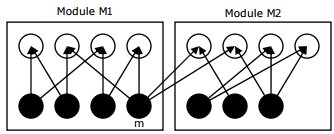Explaination / Solution: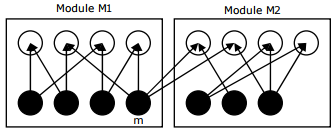Coupling = number of external links/number of modules = 2/2
Cohesion of a module = number of internal links/number of methods
Cohesion of M1 = 8/4; Cohesion of M2 = 6/3; Average cohesion=2
After moving method m to M2, graph will become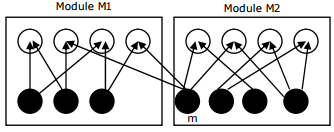Coupling = 2/2
Cohesion of M1 = 6/3; Cohesion of M2 = 8/4; Average cohesion=2

Q8. Consider a hard disk with 16 recording surfaces (0 - 15) having 16384 cylinders (0 - 16383) and each cylinder contains 64 sectors (0 - 63) . Data storage capacity in each sector is 512 bytes. Data are organized cylinder–wise and the addressing format is . A file of size 42797 KB is stored in the disk and the starting disk location of the file is <1200, 9, 40>. What is the cylinder number of the last sector of the file, if it is stored in a contiguous manner?
Explaination / Solution:

42797 KB = (42797 × 1024)/512 = 85594 sectors
Starting is (1200,9,40) contains total 24 + (6×64)=408 sectors
Next, 1201, --------, 1283 cylinders contains total 1024×83 = 84992 sec tors
each cylinder contains 16×64 = 1024 sec tors)
Total = 408+84992 = 85400 sec tors
The required cylinder number is (1284) which will contain the last sector of the file

Q9. A computer uses 46–bit virtual address, 32–bit physical address, and a three–level paged page table organization. The page table base register stores the base address of the first–level table (T1) ,which occupies exactly one page. Each entry of T1 stores the base address of a page of the second–level table (T2) Each entry of T2  stores the base address of a page of the third–level table (T3)  Each entry of T3  stores a page table entry (PTE). The PTE is 32 bits in size. The processor used in the computer has a 1 MB 16 way set associative virtually indexed physically tagged cache. The cache block size is 64 bytes.
What is the size of a page in KB in this computer?
Explaination / Solution:

Let the page size be 2x Bytes.
Then, the page offset = X bitsNow, we are using 3-level paging. First level page table is contained in one page. Each page table entry is 32-bit.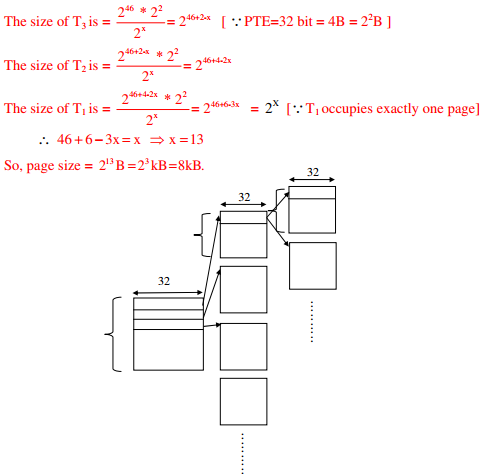Q10. A computer uses 46–bit virtual address, 32–bit physical address, and a three–level paged page table organization. The page table base register stores the base address of the first–level table (T1) ,which occupies exactly one page. Each entry of T1 stores the base address of a page of the second–level table (T2) Each entry of T2  stores the base address of a page of the third–level table (T3)  Each entry of T3  stores a page table entry (PTE). The PTE is 32 bits in size. The processor used in the computer has a 1 MB 16 way set associative virtually indexed physically tagged cache. The cache block size is 64 bytes.
What is the minimum number of page colours needed to guarantee that no two synonyms map to different sets in the processor cache of this computer?
Explaination / Solution:

As the page size is 213 Bytes and page coloring is asked so we divide cache size by page size and group 16 pages in one set.
Number of pages in cache=1MB/8KB=128 pages
Number of set in cache=128/16=8 sets
Take any page of LAS, it will be mapped with cache on any one of these 8 sets (set association mapping).For any two synonym to map with same set they should be colored with same color of that respective set. So minimum we need 8 colors for this mapping.